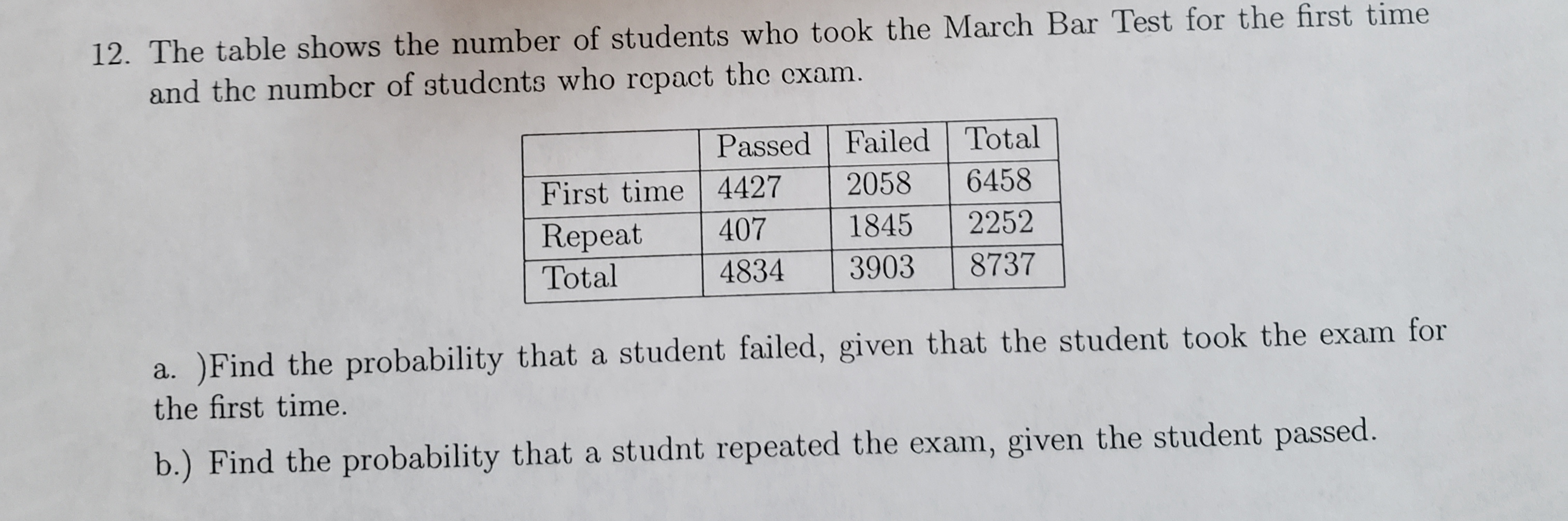# 12. The table shows the number of students who took the March Bar Test for the first timeand thc numbcr of students who repact the exam.Passed Failed Total64582058First time 442722521845407RepeatTotal873748343903a. )Find the probability that a student failed, given that the student took the exam forthe first time.b.) Find the probability that a studnt repeated the exam, given the student passed.

Question
2 viewshelp_outlineImage Transcriptionclose12. The table shows the number of students who took the March Bar Test for the first time and thc numbcr of students who repact the exam. Passed Failed Total 6458 2058 First time 4427 2252 1845 407 Repeat Total 8737 4834 3903 a. )Find the probability that a student failed, given that the student took the exam for the first time. b.) Find the probability that a studnt repeated the exam, given the student passed. fullscreen
check_circle

Step 1

Part (a)

The total number of the student is 8737.

Find the probability that a student failed, given that the student took exam for the first time.

Here we take FT is for first time having exam and R is for repeat the exam.

P is for passed student and F is for failed.

Figure out from the table. The intersection of fai...

### Want to see the full answer?

See Solution

#### Want to see this answer and more?

Solutions are written by subject experts who are available 24/7. Questions are typically answered within 1 hour.*

See Solution
*Response times may vary by subject and question.
Tagged in

### Probability